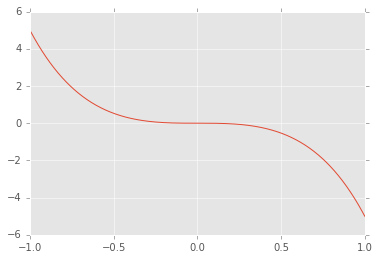# Overview¶

• Interactive programming?
• Jupyter Notebook: (more than?) a better REPL
• fast numerical arrays using numpy

Tabular data with pandas - next time?

## What do we want to be able to do?¶

• Quickly import from CSV / XLSX / DB (/clipboard?)
• Clean/pre-process/validate data
• Resample
• Aggregate
• Map/filter
• Relational operations
• Farm out tasks to R/Julia/Cython/extension modules
• Visualise
• Create narrative/audit trail (inc. markup, equations, dynamic figs)
• Export analysis

# Part 1: Interactive Python work:¶

Could use

• script + debugger
• no audit trace
• limited as to what can define
• limited in what can display

But what if want to see intermediary/final results that cannot easily be appreciated as text? e.g.

• images (e.g. heatmaps)
• graphs
• geospatial data
• mathematical expressions
• tables
  ^
|       x
y |   x x        YUK  ('~')
| x
|________>
x

Why not use browser as a REPL?

Browsers can present bitmap & vector images, tables, maths (using MathJax), video, audio...

# Ipython Notebook (now Project Jupyter)¶

Work interactively with Python (or R/Julia/Octave/JS/...) in the browser!

• Each Notebook file consists of a list of cells.
• Each cell is
• code
• Markdown/HTML (inc $LaTeX$) or
• raw (for conversion)
• Cells can contain multiple lines
• Cell only executed when done with editing (c.f. console-based REPL)
• If last block in cell is expr, display repr of that
• Can run cells in any order

Some LaTeX maths, rendered in a **Markdown** cell:

$y(x) = x^3 + \frac(x^2,3) - 6 \cdot x + 4$

Some LaTeX maths, rendered in a Markdown cell:

$y(x) = x^3 + \frac{x^2}{3} - 6 \cdot x + 4$

In :
import numpy as np
def f(a, b):
return 20 - ((a**2 - (10 * np.cos(2 * np.pi * a))) +
(b**2 - (10 * np.cos(2 * np.pi * b))))
f(3, 4)
f(5, 6)

Out:
-21.0
In :
import numpy as np
import matplotlib.pyplot as plt
plt.style.use('ggplot')
%matplotlib inline

x = np.linspace(-1, 1, 1000)
y = -x**5 - 4 * x**3

fig = plt.figure()
ax.plot(x, y)

Out:
[<matplotlib.lines.Line2D at 0x7ff52a44af28>]# Jupyter Notebok repr methods¶

Special _repr_*_ methods:

In :
class Circle(object):
raise ValueError('Must have 0 < radius <= 50')
else:
self.color = color_str

def _repr_html_(self):
return """<svg height="100" width="100">
<circle cx="50" cy="50" r="{}" fill="{}" />
Sorry, your browser does not support inline SVG.

Circle(31.2, 'red')

Out:
In :
from IPython.display import display
display(Circle(15, 'blue'))
display(Circle(31.2, 'green'))


Many projects now including _repr_html_ and _repr_latex_ methods for Ipython notebook:

• sympy for analytical maths
• pandas for manipulating tabular data
• matplotlib/bokeh/seaborn for plotting

# Grand vision¶

• Demonstrate concepts/analysis using reproducible narratives
• Validate the findings of others
• Tutorials/lectures
• Quick SA
• Text, maths, code and figures all in one place.
• Share
• as ipynb file
• via server (e.g. Nature)
• via nbviewer.ipython.org as static HTML
• document (as file) using nbconvert utility (generate HTML, PDF or LaTeX)
• as presentation (reveal.js)

# Architecture¶

### Server¶

Kernel + tornado (async web server like Node.js)

### Client¶

browser + CodeMirror text editor + MathJax (typically from CDN)

### Comms¶

ZeroMQ over websockets

## Other cool stuff¶

• ipyparallel
• cell magics: call out to other languages, create Python extension modules on the fly etc.
• shell access
• modal vim-like editing/movement!

# Part 2: Tools for interactive data manipulation¶

Much data in engineering/science/economics is array / tabular / matrix

• frequent, regular pressure measurements (array of float pressure (and timestamps?))
• river flow at different distances along a reach (array of float flows and float distances)
• discrete, irregular samples of concentration along an open channel
• data on all pipes managed by a water company (homogeneous)

How best to access/manipulate such data interactively?

• Lists/tuples are slow
• Data may be homogeneous but Python doesn't know that
• Can't use pointer arithmetic: instead iterate over array of objects of arbitrary size
• Also, cannot force lists of lists to be rectangular without encapsulation

### Numpy arrays¶

• Homogeneously typed arrays – fast creation and element access!
• C-like arrays implemented using (compiled) Python extension modules
• but with
• metadata inc datatype and size/shape
• garbage collection!
In :
import numpy as np
a = np.array([[0, 1], [1, 0]])
a

Out:
array([[0, 1],
[1, 0]])
In :
b = np.linspace(0.3, 8.9, 10)
b

Out:
array([ 0.3       ,  1.25555556,  2.21111111,  3.16666667,  4.12222222,
5.07777778,  6.03333333,  6.98888889,  7.94444444,  8.9       ])
In :
a.dtype, b.dtype

Out:
(dtype('int64'), dtype('float64'))
In :
a.shape, b.shape

Out:
((2, 2), (10,))

### Array-level operations (in C) are much faster!¶

In :
x_max = 10000
x1 = list(range(x_max))
x2 = np.arange(x_max)
%timeit [x + 1 for x in x1]
%timeit x2 + 1

1000 loops, best of 3: 746 µs per loop
The slowest run took 23.73 times longer than the fastest. This could mean that an intermediate result is being cached.
100000 loops, best of 3: 6.07 µs per loop


## Array-level operations¶

Note that many standard operators (arithmetic, comparison, element access, etc) allow for array/slice (view)-level operations.

Fast as implemented in C.

In :
distances = np.linspace(0, 1774, 50)
distances.shape, distances.max()

Out:
((50,), 1774.0)
In :
concentrations = np.random.normal(5, 3, len(distances))
concentrations.shape, concentrations.min()

Out:
((50,), 0.29912674162937236)

Ensure all concentrations are strictly positive:

In :
concentrations[concentrations < 0] = 0


Find the median concentration over all but the first and last 100 units of distance:

In :
np.median(concentrations[(distances > 100 ) & (distances <= distances[-1] - 100)])

Out:
6.1252944385858328

# Copies vs views¶

A word of warning:

• Some slicing/indexing operations return views
• modifying a view also changes the base array
• More complex slicing/indexing returns copies
• See numpy docs for rules

# Also in numpy¶

• operate on arrays/scalars of different shapes if rules regarding number of rows/columns/elements satisfied
• ufuncs
• functions where one or more arguments can be arrays
• broadcasting used to determine how to combine arguments
• vectorized wrapper for function that takes only scalar as arguments